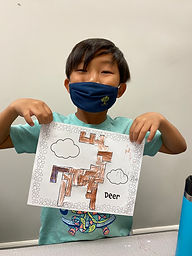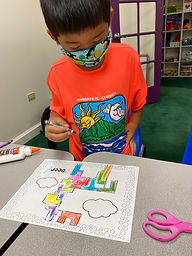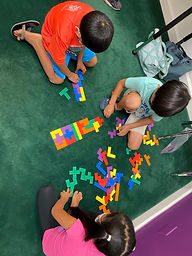## Ms. Brianne

### Target 1​

###### Lesson Type:

Continuation

Number Operation

:

Computation

Multiply within 100.

###### 1:

Understand that the same process is utilized to multiply 3 or more whole numbers that is used to multiply only two numbers (i.e., 2x3x4 = 2 groups of 3 in 6, and 4 groups of 6 is 24).

3rd

###### Vocabulary:

Product, Commutative Property, Array, Repeated Addition

Activities:

• We reviewed the commutative property, arrays, and using repeated addition or equal groups to solve multiplication problems with two numbers.
• Students selected 3 cards to make a multiplication equation. We discussed a strategy for multiplying the 3 numbers: Multiply the first and second numbers, and then multiply the product by the third number. We also talked about switching the order of the 3 numbers for easier computation.### Home Exploration

###### Guiding Questions:## Absent Students:

### Target 2

:

###### 1:

Solve pentomino puzzles by recognizing which spaces in the puzzle the given 12 pieces can and cannot fit.

###### 2:

Recognize and apply reflections (flips), translations (slides), and rotations (turns).

3rd

###### Vocabulary:

Pentomino, Flip, Slide, Turn

Activities:

• Students fit pentominoes together to create rectangles with no spaces in between the pieces.
• Students used 12 pentominoes to completely fill in the outline of a deer. They had to use flips, slides, and turns to try and fit the pieces together.### Home Exploration

###### Guiding Questions:### Target 3

:

###### Vocabulary:

Activities:### Home Exploration